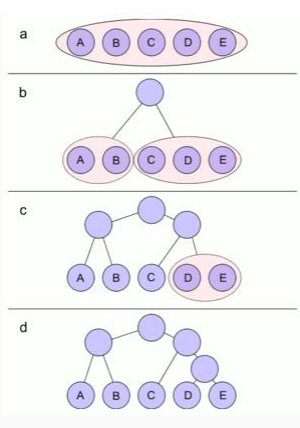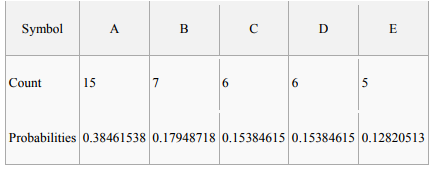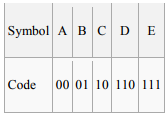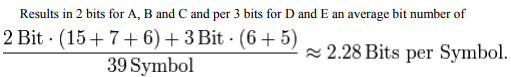Courses

# Shannon Fano Coding Electronics and Communication Engineering (ECE) Notes | EduRev

## Communication Theory

Created by: Machine Experts

## Electronics and Communication Engineering (ECE) : Shannon Fano Coding Electronics and Communication Engineering (ECE) Notes | EduRev

The document Shannon Fano Coding Electronics and Communication Engineering (ECE) Notes | EduRev is a part of the Electronics and Communication Engineering (ECE) Course Communication Theory.
All you need of Electronics and Communication Engineering (ECE) at this link: Electronics and Communication Engineering (ECE)

Shannon–Fano coding

In the field of data compression, Shannon–Fano coding, named after Claude Elwood Shannon and Robert Fano, is a technique for constructing a prefix code based on a set of symbols and their probabilities (estimated or measured). It is suboptimal in the sense that it does not achieve the lowest possible expected code word length like Huffman coding; however unlike Huffman coding, it does guarantee that all code word lengths are within one bit of their theoretical ideal − logP(x). The technique was proposed in Shannon's "A Mathematical Theory of Communication", his 1948 article introducing the field of information theory. The method was attributed to Fano, who later published it as a technical report. Shannon–Fano coding should not be confused with Shannon coding, the coding method used to prove Shannon's noiseless coding theorem, or with Shannon-Fano-Elias coding (also known as Elias coding), the precursor to arithmetic coding.

In Shannon–Fano coding, the symbols are arranged in order from most probable to least probable, and then divided into two sets whose total probabilities are as close as possible to being equal. All symbols then have the first digits of their codes assigned; symbols in the first set receive "0" and symbols in the second set receive "1". As long as any sets with more than one member remain, the same process is repeated on those sets, to determine successive digits of their codes. When a set has been reduced to one symbol, of course, this means the symbol's code is complete and will not form the prefix of any other symbol's code.

The algorithm works, and it produces fairly efficient variable-length encodings; when the two smaller sets produced by a partitioning are in fact of equal probability, the one bit of information used to distinguish them is used most efficiently. Unfortunately, Shannon–Fano does not always produce optimal prefix codes; the set of probabilities {0.35, 0.17, 0.17, 0.16, 0.15} is an example of one that will be assigned non-optimal codes by Shannon–Fano coding. For this reason, Shannon–Fano is almost never used; Huffman coding is almost as computationally simple and produces prefix codes that always achieve the lowest expected code word length, under the constraints that each symbol is represented by a code formed of an integral number of bits. This is a constraint that is often unneeded, since the codes will be packed end-to-end in long sequences. If we consider groups of codes at a time, symbol-by-symbol Huffman coding is only optimal if the probabilities of the symbols are independent and are some power of a half, i.e., . In most situations, arithmetic coding can produce greater overall compression than either Huffman or Shannon– Fano, since it can encode in fractional numbers of bits which more closely approximate the actual information content of the symbol. However, arithmetic coding has not superseded Huffman the way that Huffman supersedes Shannon–Fano, both because arithmetic coding is more computationally expensive and because it is covered by multiple patents. Shannon–Fano coding is used in the IMPLODE compression method, which is part of the ZIP file format.

SHANNON–FANO ALGORITHM:

A Shannon–Fano tree is built according to a specification designed to define an effective code table. The actual algorithm is simple:

1. For a given list of symbols, develop a corresponding list of probabilities or frequency counts so that each symbol‘s relative frequency of occurrence is known.

2. Sort the lists of symbols according to frequency, with the most frequently occurring symbols at the left and the least common at the right.

3. Divide the list into two parts, with the total frequency counts of the left half being as close to the total of the right as possible.

4. The left half of the list is assigned the binary digit 0, and the right half is assigned the digit 1. This means that the codes for the symbols in the first half will all start with 0, and the codes in the second half will all start with 1.

5. Recursively apply the steps 3 and 4 to each of the two halves, subdividing groups and adding bits to the codes until each symbol has become a corresponding code leaf on the tree.Shannon–Fano Algorithm

The example shows the construction of the Shannon code for a small alphabet. The five symbols which can be coded have the following frequency:All symbols are sorted by frequency, from left to right (shown in Figure a). Putting the dividing line between symbols B and C results in a total of 22 in the left group and a total of 17 in the right group.

This minimizes the difference in totals between the two groups.

With this division, A and B will each have a code that starts with a 0 bit, and the C, D, and E codes will all start with a 1, as shown in Figure b. Subsequently, the left half of the tree gets a new division between A and B, which puts A on a leaf with code 00 and B on a leaf with code 01.

After four division procedures, a tree of codes results. In the final tree, the three symbols with the highest frequencies have all been assigned 2-bit codes, and two symbols with lower counts have 3-bit codes as shown table below:Offer running on EduRev: Apply code STAYHOME200 to get INR 200 off on our premium plan EduRev Infinity!

,

,

,

,

,

,

,

,

,

,

,

,

,

,

,

,

,

,

,

,

,

;